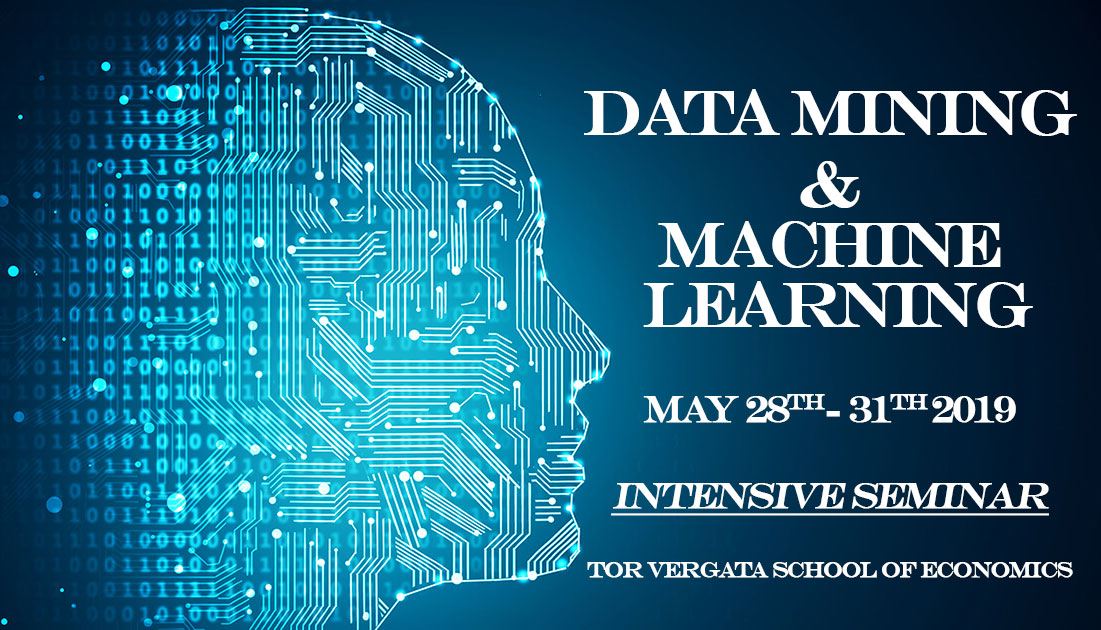### Data Mining/Machine Learning seminar (3 CFU) - 2° module

A strong focus on machine learning, regression techniques, evaluation metrics, decision trees, ensemble learning, clustering algorithms.The seminar in presented by Mauro Castelli, Assistant Professor at NOVA IMS (Universidade NOVA de Lisboa, Portugal) and Illya Bakurov, PhD student at NOVA IMS (Universidade NOVA de Lisboa, Portugal) in collaboration with Prof. Simone Borra.

# COURSE STRUCTURE

### DAY 1

Basic concepts about machine learning (ML). The general idea of machine learning is introduced and a formal definition is provided. Several applications where ML is used in our daily life are presented to allow students to fully understand the breakthrough provided by this discipline. Subsequently, the concepts of training set, test set, and features are introduced and the differences between supervised and unsupervised learning are formally defined.
The second part of the class formalizes the general statement of a regression problem, where the objective is to predict a real-valued output based on one or more input values. Subsequently, some of the most commonly used regression techniques are presented. The general statement of a classification problem is introduced, highlighting its main differences with a regression problem.

### DAY 2

Logistic regression as wells as common loss functions for classification problems. Subsequently, the concept of confusion matrix is introduced, as well as different evaluation metrics (i.e., accuracy, recall, F-measure, AUROC). The selection of the evaluation metric in the case of unbalanced datasets is also discussed.
In the final part of the class, model selection will be covered. As a first step, the concepts of approximation and estimation errors are introduced. This allows presenting an important problem that affects several ML techniques: overfitting. Finally, the concept of K-fold cross-validation is introduced, followed by a discussion about its importance for guaranteeing that robust and reliable models are generated.

### DAY 3

Decision trees, a supervised ML technique that can be used to solve both regression and classification problems. The explanation considers the case of a classification problem, but the concepts are then extended to regression tasks.
The second part of the class discusses ensemble learning. This concept is introduced by considering several real-world problems, to provide an intuitive understanding of the properties of ensemble models.
Subsequently, Random Forest, an ensemble model that consists of different decision trees, is presented. The presentation is followed by a discussion about ensemble diversity, bias/variance trade-off, and overfitting.

### DAY 4

This class is dedicated to dimensionality reduction techniques. Subsequently, different dimensionality reduction techniques are presented.
The second part of the class introduces the general idea of clustering (i.e., to group unlabeled data points such that “similar” points will be assigned to the same cluster) by considering several examples. After this overview of clustering techniques, the K-means algorithm is introduced.  Subsequently, hierarchical clustering is presented and the differences between agglomerative and divisive algorithms are discussed.

# TIMETABLE

 DAY TIME ROOM 28.05.2019 10:00 - 13:00           14:00 - 17:00 S4 29.05.2019 10:00 - 13:00           14:00 - 17:00 S4 30.05.2019 10:00 - 13:00           14:00 - 17:00 S4 31.05.2019 10:00 - 13:00           14:00 - 17:00 S4

# REGISTRATION

Students who are interested in participating are required to complete the form.